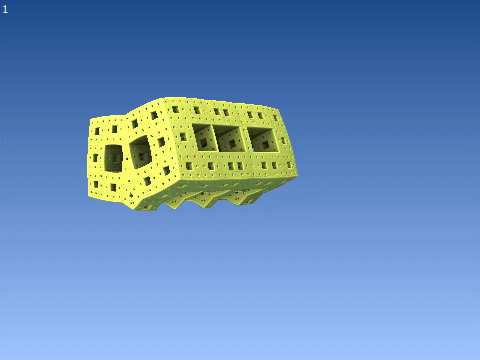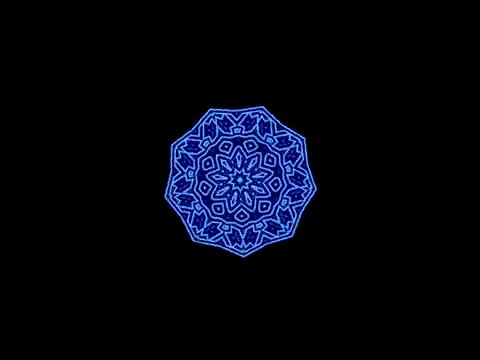1. ## Symmetry Demos

I have a page, Symmetries, a page that links to several demos of space symmetries that I have created.

Playing-Card Symmetries is about the symmetries of standard playing cards.

Frieze Groups, Wallpaper Groups are done in ASCII art.

2D Point-Group Demo makes a regular polygon, and you can then rotate and reflect it. Organism-Symmetry Demo does the same with pictures of various organisms and organism parts.

Organism-Symmetry Gallery shows lots of pictures of organisms with various symmetries. Bilateral, linear, plane-grid, polyhedral, and fractal, among others.Reply With Quote

2. I did symmetries a while back too. Different toy set (something I've tried to get Loren into).

Anyway. These use the exact same symmetry operations. There are various symmetry relations I ran into in 3 dimensions that you don't run into in 2. Which Loren can describe with words. I can show you pictures, but it takes more work.Reply With Quote

3. What symmetries are these videos intended to demonstrate? I can recognize the rosette groups and the Menger sponge, for instance.

Here are the seven frieze groups done as ASCII art:
Code:
p1 C1
b b b b

p1m D1
b b b b
p p p p

p1g D1
b b b b
p p p p

pm1 D1
bdbdbdbd

p2 C2
b b b b
q q q q

pmm D2
bdbdbdbd
pqpqpqpq

pmg D2
bdbdbdbd
qpqpqpqp
Each one is listed with its associated point group.

Here are some of the seventeen wallpaper groups' unit cells done as ASCII art:
Code:
p1 C1
b

p2 C2
bq

pm D1
bd

pg D1
bp

cm D1
<bd>

pmm D2
bd
pq

pmg D2
bd
qp

pgg D2
bp
dq

cmm D4
/bd\
\pq/
The remaining ones are more difficult for ASCII art -- p4 C4, p4m D4, p4g D4, p3 C3, p3m1 D3, p31m D3, p6 C6, p6m D6Reply With Quote

4. There are only two 1D point groups: C (identity) and D (reflection).

Each one has associated line groups: continuous and discrete.

There are two infinite families of 2D point groups:
C(n) -- n-fold rotation
D(n) -- C(n) + n reflections
These groups can be interpreted as 1D discrete line groups wrapped in a circle with n repeats.

There are seven infinite families of 3D point groups, essentially each frieze group wrapped in a circle. These are the axial or prismatic groups.
C(n) -- p1
C(n,h) -- p1m
S(2n) -- p1g
C(n,v) -- pm1
D(n) -- p2
D(n,h) -- pmm
D(n,d) -- pmg
where n is the number of repeats of the frieze-group unit cell.

There are seven additional 3D point groups, the quasi-spherical or polyhedral or Platonic ones.
T -- tetrahedron rotations
Th -- tetrahedron rotations and inversions (the volleyball group)
Td -- tetrahedron rotations and reflections
O -- octahedron rotations
Oh -- octahedron rotations and reflections
I -- icosahedron rotations
Ih -- icosahedron rotations and reflections

The group of all n-D rotations is SO(n) and of n-D rotations and reflections O(n).Reply With Quote

5. Rotational symmetries.

Rosette groups? That's just code I wrote that looked nice. Originally noticed it when I was working on 3d symmetries of tetrahedrons:Which came from:Reply With Quote

6. Nice workReply With Quote

7. Rosette groups = 2D point groups.

Here are that group's two types of matrices.

Rotations:

Reflections:

Full group:
SO(2): R(θ) for all values of θ between 0 and 2pi
O(2): SO(2) with S(θ) for all values of θ between 0 and 2pi.

Finite subgroups of it:
C(n): R(2pi*k/n) for k = 0 to (n-1)
D(n): C(n) with S(θ0 + 2pi*k/n) for k = 0 to (n-1) and some θ0Reply With Quote

8. Is D(n) non symmetric, or am I reading it wrong?

To make it symmetric, I'd do:

DkOdd(n) : S(θ0 (-1)k+ 2pi*k/(2n)) for k = 0 to (2n-1)

I like that better- that's what I used for non-even n (odd n), although with even n you can just do:

DkEven(n) : S(θ0 (-1)k+ 2pi*k/(n)) for k = 0 to (n-1)Reply With Quote

9. That won't work.

Here is how the rotation and reflection matrices combine:
• R(a).R(b) = R(a+b)
• R(a).S(b) = S(a+b)
• S(a).R(b) = S(a-b)
• S(a).S(b) = R(a-b)

Notice this interesting operation table:
R S
S R

Thus, there is a homomorphism or mapping of D(n) onto Z2. The kernel, the part that gets the identity, is all the R matrices. It is a "normal subgroup", and the S's together and R's together group is a "quotient group".

For D(even), we get a further one, distinguishing even and odd elements:
Re Ro Se So
Ro Re So Se
Se So Re Ro
So Se Ro Re

This is the group Z2*Z2.Reply With Quote

10.Originally Posted by lpetrichThat won't work.
lol... sure.Reply With Quote

####Posting Permissions

• You may not post new threads
• You may not post replies
• You may not post attachments
• You may not edit your posts
•